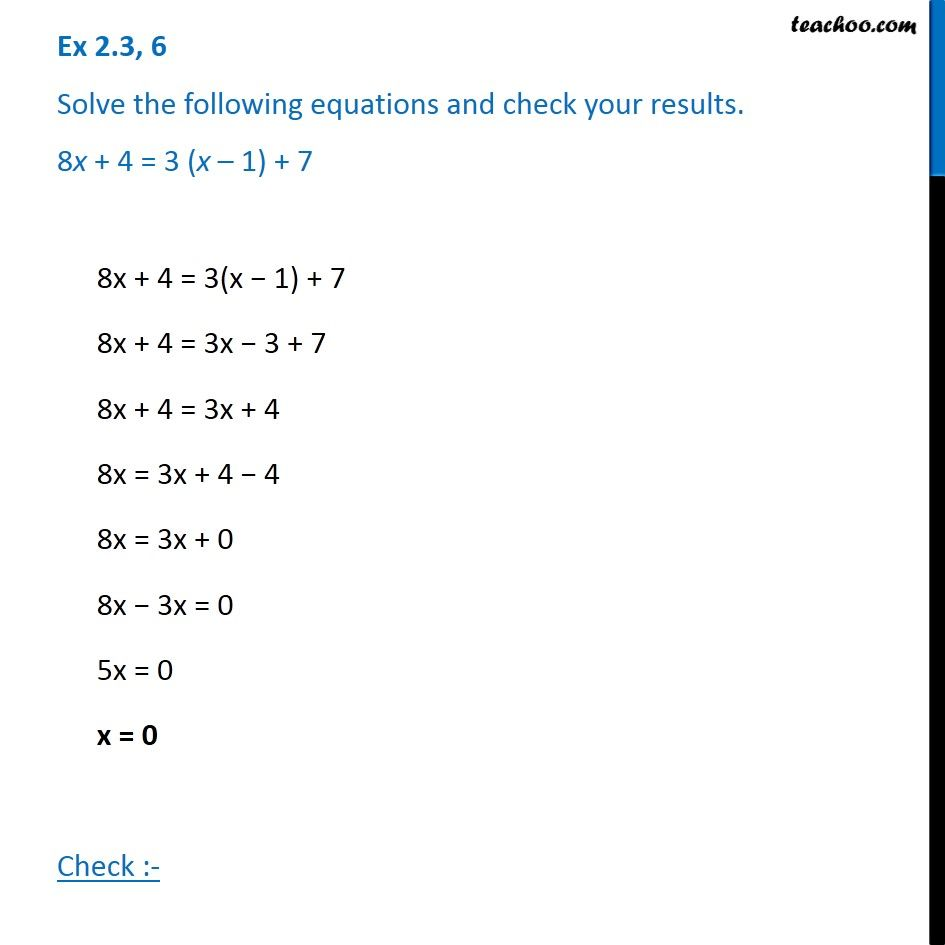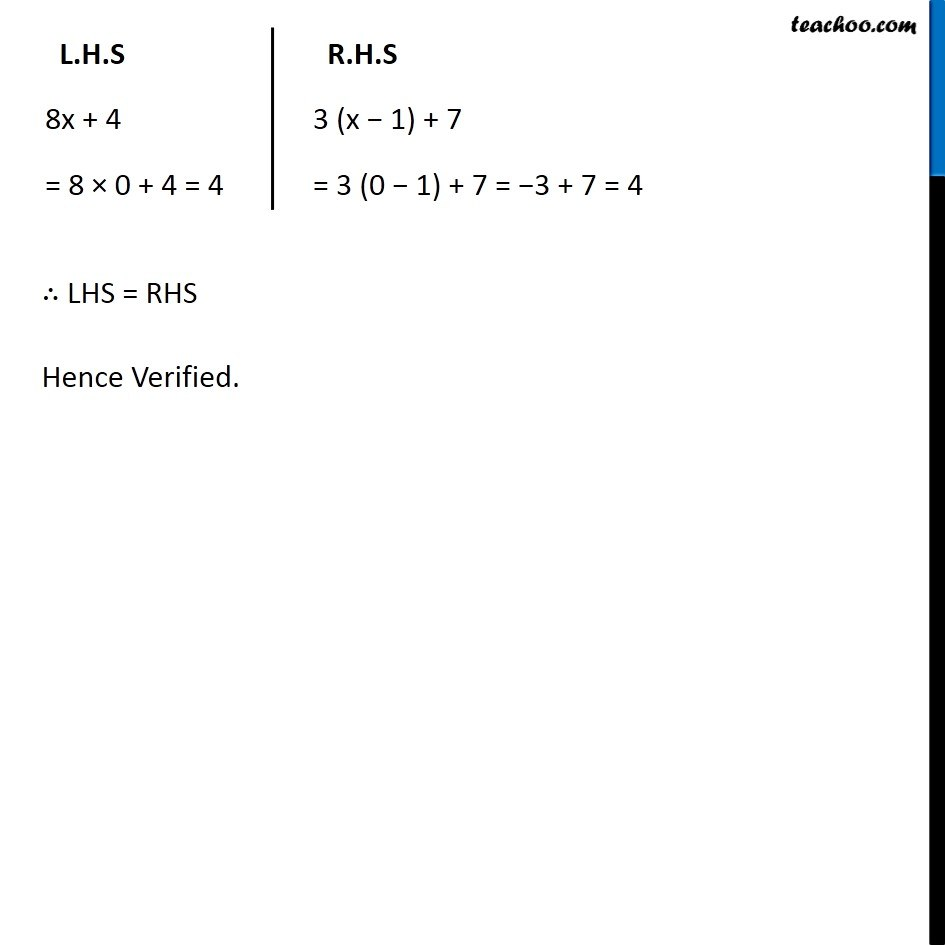Ex 2.3

Chapter 2 Class 8 Linear Equations in One Variable
Serial order wiseGet live Maths 1-on-1 Classs - Class 6 to 12

### Transcript

Ex 2.3, 6 Solve the following equations and check your results. 8x + 4 = 3 (x – 1) + 78x + 4 = 3(x − 1) + 7 8x + 4 = 3x − 3 + 7 8x + 4 = 3x + 4 8x = 3x + 4 − 4 8x = 3x + 0 8x − 3x = 0 5x = 0 x = 0 Check :- L.H.S 8x + 4 = 8 × 0 + 4 = 4 R.H.S 3 (x − 1) + 7 = 3 (0 − 1) + 7 = −3 + 7 = 4 ∴ LHS = RHS Hence Verified.